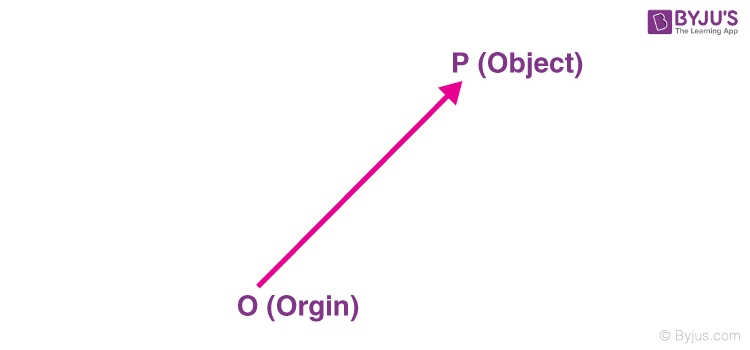# Position And Displacement Vectors

One of the primary things to start with Kinematics is to know what position vector is, what displacement vector is, and the difference between the position vector and displacement vector. Let us learn about position vectors in detail in this article.

## What is a Position Vector?

The position vector is used to specify the position of a certain body. Knowing the position of a body is vital when describing the motion of that body.

### Position Vector Definition

A position vector is defined as a vector that symbolises either the position or the location of any given point with respect to any arbitrary reference point like the origin. The direction of the position vector always points from the origin of that vector towards a given point.

### Position Vector Example

The position vector of an object is measured from the origin, in general. Suppose an object is placed in the space as shown:Position vector

$$\begin{array}{l}(\vec{r})=x\hat{i}+y\hat{j}+z\hat{k}\end{array}$$

Where,

$$\begin{array}{l}\hat{i}= unit\,vector\,along\,x-direction\end{array}$$
$$\begin{array}{l}\hat{j}= unit\,vector\,along\,y-direction\end{array}$$
$$\begin{array}{l}\hat{k}= unit\,vector\,along\,z-direction\end{array}$$

### Position Vector Formula

To find the position vector of any point in the xy-plane, we should first know the point coordinates. Consider two points A and B whose coordinates are (x1, y1) and (x2, y2), respectively. To determine the position vector, we need to subtract the corresponding components of A from B as follows:

AB = (x2 – x1) i + (y2 – y1) j

The position vector AB originates from point A and terminates at point B.

### What is a Displacement Vector?

The change in the position vector of an object is known as the displacement vector. Suppose an object is at point A at time = 0 and at point B at time = t. The position vectors of the object at point A and point B are given as:

Position vector at point

$$\begin{array}{l}A=\hat{r_{A}}=5\hat{i}+3\hat{j}+4\hat{k}\end{array}$$

Position vector at point

$$\begin{array}{l}B=\hat{r_{B}}=2\hat{i}+2\hat{j}+1\hat{k}\end{array}$$

Now, the displacement vector of the object from time interval 0 to t will be:

$$\begin{array}{l}\hat{r_{B}}-\hat{r_{B}}=-3\hat{i}-\hat{j}-3\hat{k}\end{array}$$

The displacement of an object can also be defined as the vector distance between the initial point and the final point. Suppose an object travels from point A to point B in the path shown in the black curve:The displacement of the particle would be the vector line AB, headed in the direction A to B. The direction of the displacement vector is always headed from the initial point to the final point.

## Frequently Asked Questions – FAQs

Q1

### What is a position vector?

Vectors that specify the position of the body are known as position vectors. Often they start at the origin and terminate at an arbitrary point.
Q2

### Is velocity a position vector?

Displacement is the vector difference between the ending and starting positions of an object.
Q3

### What is a displacement vector?

A displacement vector tells you how much the object’s position has changed. The displacement vector includes how far you have travelled and in which direction you have travelled.
Q4

### What is the formula of position vector?

The position vector of points A and B is AB = (x2 – x1, y2 – y1) = (x2 – x1) i + (y2 – y1) j
Q5

### What does position vector represent?

A position vector is defined as a vector that indicates either the position or the location of any given point with respect to any arbitrary reference point like the origin.

## Polygon law of vector addition states that if every side of a polygon represents a vector, their sum taken in cyclic order is zero. Watch the video to learn more.Stay tuned with BYJU’S to know more about the scalar product, dot products, and much more with exciting video lectures and fall in love with learning.

Test your Knowledge on Position And Displacement Vectors